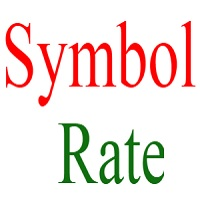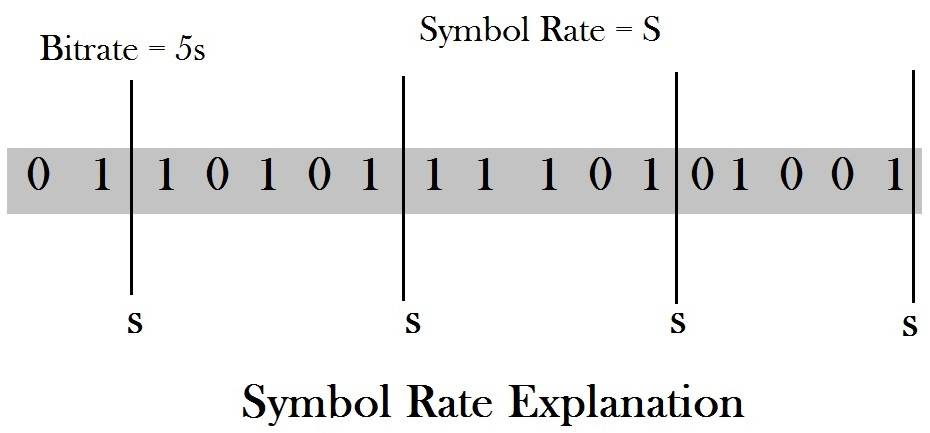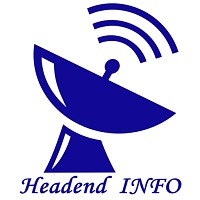# What Is Symbol Rate Or Baud Rate For DVB Or Digital Video Broadcasting## Introduction

Whenever a headend engineer works in Digital Headend then gets in contact with terms Symbol Rate , Bit Rate , Frequency , Modulation and FEC. These terms comes under tuning parameters.

Generally we see these terms in IRDs and QAMs.

In this article we will particularly focus on Symbol Rate (SR) that What Is SR , how it is implemented and why SR is necessary for Digital Video Broadcasting.## What Is Symbol Rate Or Baud Rate

A Symbol Rate is also known as Baud Rate. The word Baud came from French Engineer named as Emile Baudot. Emile Baudot invented 5 bit telegraphic code.

A Symbol Rate is number of symbol changes in second or in a unit of time. Whereas a symbol is made of several voltages , phase and frequency changes.

In Other Words,

A Symbol Rate is known as how many symbols we are transmitting per second in communication channel. So SR is measured in symbols per second.

Every symbol consist of fixed number of bits. These number of bits can be 1 bit , 2 bit 3 bit or more.

As we know binary signals are in the form of 0 or 1. We can not transmit baseband binary signals directly for this we need to use modulation technique. For modulation we need to super impose our signal on the high frequency carrier wave. This process also increase the data rate. So Cable tv companies use modulation techniques to transmit their signals at long distance with high data rate.As shown above in the figure Symbol Rate represent S while bitrate represent the 5 times S. Because as we can see in the figure each symbol rate contains 5 bits.

keep in mind one thing the each symbol contains fixed number of bits. These fixed number of bits can be 1 bit , 2 bit or 3 bit for each symbol. Thus symbol rate defined as number of symbols per second in a communication channel.

8VSB is used in United States in HDTV modulation. 8VSB is known as 8 level Vestigial Sideband. In each symbol it contains 3 bits. In the transmission the SR is 10,800 Symbols per second. So its bitrate can be calculated as 3 * 10800 = 32.4 Mbps.

## Conclusion

A Symbol Rate is also known as Baud Rate. SR is used for the calculation of Bitrate of a communication channel. SR is a tuning parameter for transmission of signal. SR is defined by the character “S”. It is counted by total number of symbol available in a transmission.

## Know More About   Tuning Of Digital Signal Transmission Devices